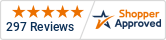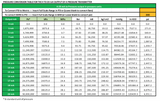Intro to Pressure Units

Free Shipping! - USA Orders Over \$75# Intro to Pressure Units

The Origin of Pressure Units

All units were originally derived from readily available physical standards that laboratories around the world could duplicate. The Fahrenheit temperature scale was set with 0° was the coldest you could get an ice salt slurry and 100° was body temperature. The Celsius Temperature scale was 0° at the freezing point of water and 100° at the boiling point of water. Pressure is a more complex parameter defined as “force per unit area”.

In England and America, the unit of force was the pound and the unit for area was square inch, hence, the Pressure unit became pounds per square inch (psi). In this system, Atmospheric pressure was 14.7 psi at sea level. On Continental Europe, the unit of weight is the gram and the unit for area the meter, but g/m2 was not a workable unit so kg/cm2 was used. Atmospheric pressure was determined and given the unit BAR (1 BAR = 14.5 psi). This is an interesting anomaly, I do not know the background but suspect measurement “accuracy” at the time such as with Fahrenheit determining body temperature to be 100° rather than what we now today is actually 98.6°.

Temperature and pressure are certain foundational units that are enshrined in everyone’s daily life. That makes them difficult to change. Here in the United States, we differ from the rest of the world by holding onto the pre-metric (English) units in public life. We still use the Fahrenheit system for temperature, inch/foot/yard/mile units for length, cup, pint, quart, gallon for volume, miles per hour for velocity, and pounds per square inch for pressure. When I went to engineering school, we learned both sets of units (English and Metric) and could readily convert between them in calculations; but in public life, the metric system never caught on in America. How could you build a house without 2x4x8's or describe temperature with 32° Hot in °Celsius or Cold in °Fahrenheit? The same seems true for velocity/speed (although if 60 km/hr was faster than 60 mph it might have caught on). Pressure also seems to be an especially difficult unit to cross to metric. Atmospheric pressure is 14.7 psi at sea level whereas the metric system uses the BAR for atmospheric pressure and 1 BAR is equal to only 14.5 psi. Adding to the confusion is the fact that the metric world used several different units for pressure. Some countries used the BAR, others used kg/cm2 where 1 kg/cm2 = 14.22 psi and 0.98 BAR. For low pressures  and vacuum measurements "inches of water" was used in America and TORR (mm of Mercury) was used in the European system. With all these units based on different physical parameters,  there was never a clean conversion from the  American/English pressure units to the European/metric equivalent units. Now the European community has standardized on the SI (System International) system of measurement with the unit for pressure being the Pascal (Pa).

What is a PASCAL?

The Pascal is the defined unit of measure for Pressure in the SI metric system of measurement and is defined as 1 Newton/meter2.  A Newton is a force that will give a mass of 1kg an acceleration of 1m/s2 .  In the American/English system a Newton is about 0.225 lb and a square meter is 10.76 sq ft, so 1 psi = 6895 pascals or 6.8 kPa.

How does a Pascal relate to pre-existing units?

1 pascal is equal to 0.00014503773800722 psi. As you can see, the Pascal is a very small unit in the practical world. As such, the kPa (1,000 Pa) and MPa (1,000,000 Pa) units are also commonly used.

1 kPa = 0.145 psi and 1 MPa = 145 psi

Atmosphere Pressure (14.5 psi in the metric system) equates to 0.1 MPa, 1 Bar, 100 kPa, and 99,974 Pa.
1 psi in Pascals is 6,895 Pa and 6.9 kPa; (in very round numbers 1 psi = 7 kPa). There is nothing there that is easy for the common population to relate to. In industry, 3-15 psi control air now becomes 20 to 103 kPa and 100 psi becomes 689 kPa.

Many excellent pressure transducers manufactured in the metric world and calibrated and scaled in metric units, typically BARs, are becoming more popular in the US. These transducers are plug-in compatible electrically but take a little bit of rescaling of your instruments. 1 BAR = 14.5 psi (15 in round numbers). A 10 Bar range is approximately 150 psi, 20 Bar 300 psi and 30 Bar 450 psi 100 BAR IS 1500 PSI…Pressure Conversion Tool

To help with scaling and setting up equipment there is a pressure conversion table that will convert Bars to psi (and most common metric units). It’s set up to show the pressure in psi at each whole mA output from a 4 to 20mA output pressure transmitter scaled in Bars. (Converting BARS to PSI). It will also work the other way, converting PSI to Bars for each mA output.Download the Spreadsheet